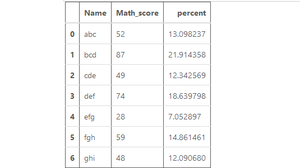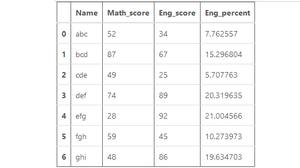# How to calculate the Percentage of a column in Pandas ?

A Percentage is calculated by the mathematical formula of dividing the value by the sum of all the values and then multiplying the sum by 100. This is also applicable in Pandas Dataframes. Here, the pre-defined sum() method of pandas series is used to compute the sum of all the values of a column.

Syntax: Series.sum()

Return: Returns the sum of the values.

Formula:

```df[percent] = (df['column_name'] / df['column_name'].sum()) * 100
```

Example 1:

## Python3

 `# Import required libraries ` `import` `pandas as pd ` `import` `numpy as np ` `  `  `# Dictionary ` `df1 ``=` `{ ` `     ``'Name'``: [``'abc'``, ``'bcd'``, ``'cde'``, ` `             ``'def'``, ``'efg'``, ``'fgh'``, ` `             ``'ghi'``], ` `     ``'Math_score'``: [``52``, ``87``, ``49``, ` `                   ``74``, ``28``, ``59``, ` `                   ``48``]} ` `  `  `# Create a DataFrame ` `df1 ``=` `pd.DataFrame(df1,  ` `                   ``columns ``=` `[``'Name'``, ` `                             ``'Math_score'``]) ` ` `  `# Calculating Percentage ` `df1[``'percent'``] ``=` `(df1[``'Math_score'``] ``/`  `                  ``df1[``'Math_score'``].``sum``()) ``*` `100` ` `  `# Show the dataframe ` `df1 `

Output:Example 2:

## Python3

 `# Import pandas library ` `import` `pandas as pd ` `  `  `# Dictionary ` `df1 ``=` `{ ` `     ``'Name'``: [``'abc'``, ``'bcd'``, ``'cde'``, ` `             ``'def'``, ``'efg'``, ``'fgh'``, ` `             ``'ghi'``], ` `      ``'Math_score'``: [``52``, ``87``, ``49``, ` `                    ``74``, ``28``, ``59``, ` `                    ``48``], ` `       ``'Eng_score'``: [``34``, ``67``, ``25``,  ` `                    ``89``, ``92``, ``45``, ` `                    ``86``] ` `} ` `  `  `# Create a DataFrame ` `df1 ``=` `pd.DataFrame(df1,  ` `                   ``columns ``=` `[``'Name'``, ` `                   ``'Math_score'``,``'Eng_score'``]) ` ` `  `# Calculate Percentage ` `df1[``'Eng_percent'``] ``=` `(df1[``'Eng_score'``] ``/`  `                      ``df1[``'Eng_score'``].``sum``()) ``*` `100` `# Show the dataframe ` `df1 `

Output:My Personal Notes arrow_drop_upCheck out this Author's contributed articles.

If you like GeeksforGeeks and would like to contribute, you can also write an article using contribute.geeksforgeeks.org or mail your article to contribute@geeksforgeeks.org. See your article appearing on the GeeksforGeeks main page and help other Geeks.

Please Improve this article if you find anything incorrect by clicking on the "Improve Article" button below.

Article Tags :

Be the First to upvote.

Please write to us at contribute@geeksforgeeks.org to report any issue with the above content.